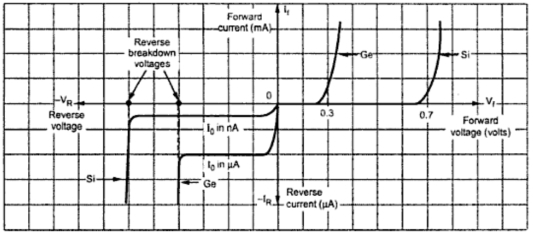### Complete V-I Characteristics of a Diode

The complete V-I characteristics of a diode is the combination of its forward as well as reverse characteristics. This is shown in the Fig. 1.Fig. 1  Complete V-I characteristics of a diode
In forward characteristics, it is seen that initially forward current is small as long as the bias voltage is less that the barrier potential. At a certain voltage close to barrier potential, current increases rapidly. The voltage at which diode current starts increasing rapidly is called as cut in voltage. It is denoted by Vγ. Below this voltage, current is less than 1% of maximum rated value of diode current. The cut-in voltage for germanium is about 0.2 V while for silicon it is 0.6 V.
It is important to note that the breakdown voltage is much higher and practically diodes are not operated in the breakdown condition. The voltage at which breakdown occurs is called reverse breakdown voltage denoted as VBR.
Note : Reverse current before the breakdown is very very small and can be practically neglected.
1.1 V-I Characteristics of Typical Ge and Si Diodes
The combined forward and reverse characteristics is called V-I characteristics of a diode. As mentioned earlier, the barrier potential for germanium (Ge) diode is about 0.3 V while for silicon (Si) diode is as about 0.7 V. The potential at which current starts increasing exponentially is also called offset potential, threshold potential or firing potential of a diode. The Fig. 2 shows the V-I characteristics of typical Ge and Si diodes.Fig. 2 V-I characteristics of typical Ge and Si diodes
The reverse saturation current in a germanium diode is about 1000 times more than the reverse saturation current in a silicon diode of a comparable rating. The reverse saturation current I0 is of the order of nA for silicon diode while it is of the order of μA for germanium diode. Reverse breakdown voltage for Si diode is higher than that of the Ge diode of a comparable rating.